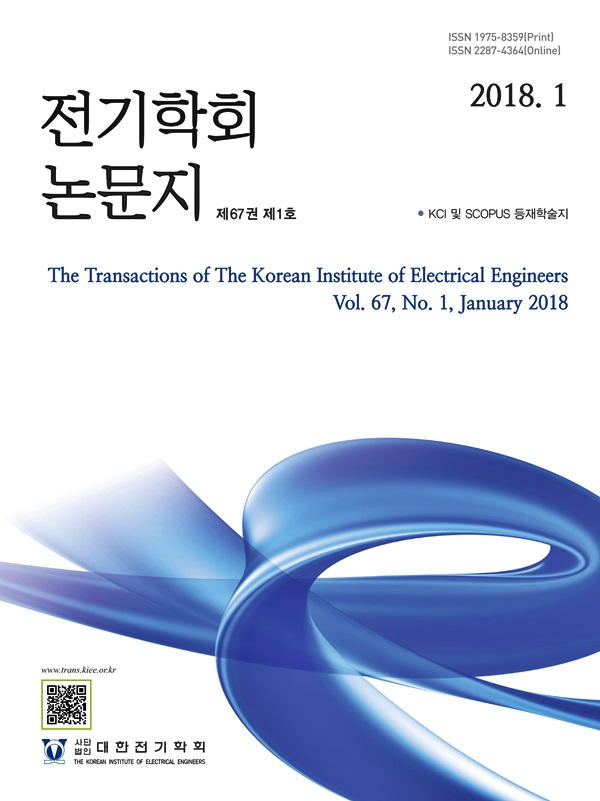Mobile QR CodeThe Transactions of the Korean Institute of Electrical Engineers

ISO Journal TitleTrans. Korean. Inst. Elect. Eng.
Title Comparative Study on Data based and Model based ESR Estimation Techniques for DC/AC Converter 박혜진(Hyejin Park) ; 곽상신(Sangshin Kwak) https://doi.org/10.5370/KIEE.2021.70.12.1862 pp.1862-1869 1975-8359 DC link electrolytic capacitor; DC/AC converter; equivalent series resistor (ESR); model based ESR estimation; data based estimation In this paper, the performance of the input capacitor state estimation algorithm of the DC/AC single-phase converter implemented using the model-based method and the data-based method is compared. The state of the capacitor is estimated through two existing algorithms implemented as a model-based method and the data based algorithm implemented as a data-based method. By applying the capacitor voltage and capacitor current data collected through the experiment to three methods, the equivalent series resistor (ESR) component was derived. The two model-based methods used in this paper are the method of estimating the ESR component using a bandpass filter and the method of using the average power loss. The data-based method estimates ESR using a component twice the fundamental frequency and a component twice the switching frequency in the frequency domain as input to the neural network. Comparing the results, it was confirmed that in the case of the model-based method, the deviation of the error according to the ESR was very large. On the other hand, in the case of the data-based method, it was confirmed that the estimation was made more accurately using data of a large amount of information. Therefore, it was confirmed that the data-based method showed excellent estimation results in all fields